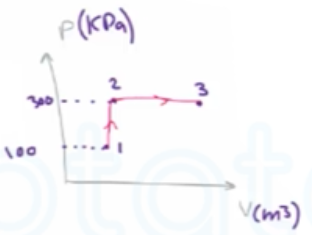Need Help?

Subscribe to Thermodynamics

###### \${selected_topic_name}
• Notes
• Comments & Questions

$\begin{array}{l}{\text { Consider a piston-cylinder device that contains nitrogen } \mathrm{gas} \text { as the system }} \\ {\text { Initially, the system is at } 1 \mathrm{MPa} \text { and } 427^{\circ} \mathrm{C} \text { . It now undergoes }} \\ {\text { an isobaric process until its temperature is } 27^{\circ} \mathrm{C} \text { . Determine }} \\ {\text { the final pressure and the heat transfer, in } \mathrm{k} \mathrm{J} / \mathrm{kg} \text { , associated }} \\ {\text { with this process. }}\end{array}$

$A-2 a \longrightarrow C_{P}=1.039 \mathrm{kJ} / \mathrm{kg} . \mathrm{k}$

$∵ \text { isobaric }:$ $P_{1}=P_{2}=1 M P_{a}$

$E_{i n}-E_{o u t}=\Delta E_{system}$

$-q_{out} -W_{b\ {o u} t}=\Delta U=\left(U_{2}-U_{1}\right)$

$\rightarrow {-q_{\text { out }}}=W_{b \ out}+(U_2-U_1)$

$\left[\Delta h=\Delta u+w_{b}\right]$

$∴-q_{out}=\Delta h=h_{2}-h_{1}$

$q_{out}=m c{p} \ \left(T_{1}-T_{2}\right)$

$=Cp(T_1-T_2)=(1.039)(472-27)=416 \ kJ/kg$

$\begin{array}{l}{\text { A piston-cylinder device containing argon } g \text { as as the system undergoes an isothermal }} \\ {\text { process from } 200 \mathrm{kPa} \text { and } 100^{\circ} \mathrm{C} \text { to } 50 \mathrm{kPa} \text { . During the process. } 1500 \mathrm{kJ} \text { of heat is }} \\ {\text { transferred to the system. Determine the mass of this system and the amount of work produced }}\end{array}$

$A-1 \longrightarrow R=0.2081 \mathrm{kJ} / \mathrm{kg}$

$P_{1}=200 \mathrm{KPa}$    $T_{1}=100 c^{\circ}$        $P_{2}=50 \mathrm{kP}$

$\text { isothermal }\longrightarrow$$T_{1}=T_{2}=100 C^{\circ}$

$E_{i n}-E_{\text { out }}=\Delta E_{\text { system }}$

$Q_{\text { in }}-W_{\text { b out }}=\Delta U=m \ c_v (T_2-T_1)$

$Q_{\text { in }}-W_ \text {b out }=0$

$\rightarrow Q_{i n}=W_{b \ o u t}=1500 \mathrm{kJ}$

$W_{b \ out}={m} \int^{2}_{1} P d v= m R T \int^{2}_{1}\frac{dv}{v}=mRT \ln\frac{v_2}{v_1}$

$W_{b \ out}={m}R T \ln\frac{P_1}{P_2}\rightarrow m=\frac{W_{b,out}}{RT\ln\frac{P_1}{P_2}}$

$\rightarrow m=\frac{1500}{0.2081^{*} 373 * \ln \frac{200}{50}}={13.94 \mathrm{kg}}$

$\begin{array}{l}{\text { Air is contained in a cylinder device fitted with a piston-cylinder. The piston initially rests on a }} \\ {\text { set of stops, and a pressure of } 300 \mathrm{kPa} \text { is required to move the piston. Initially, the air is at } 100 \mathrm{kPa}}\end{array}$

$\begin{array}{l}{\text { and } 27^{\circ} \mathrm{C} \text { and occupies a volume of } \mathrm{o} \cdot 4 \mathrm{m}_{3} \text { . Determine the amount of heat transferred to the air, in } \mathrm{kJ} \text { , }} \\ {\text { while increasing the temperature to } 1200 \mathrm{K} \text { . Assume air has constant specific heats evaluated at } 300 \mathrm{K} \text { . }}\end{array}$

$A-2 a \longrightarrow$

$R=0.287 \mathrm{KJ} / \mathrm{kg} \cdot \mathrm{K}$

$C_{V}=0.718 \mathrm{kJ} / \mathrm{kg.k}$

$E_{i n-} E_{out} =\Delta E_ \text { system }$

$Q_{i n}-W_{b \ o u t}=\Delta U=m c_{v}\left(T_{2}-T_{1}\right)$

$T_{2}=T_{1} \frac{p_{2}}{p_{1}}=300\left(\frac{300}{100}\right)=900 \mathrm{k}$

$p_{1} v_{1}=m R T_{1} \longrightarrow m$$=\frac{P_{1} v_{1}}{R T_1}=\frac{100 * 0.4}{0.287 * 300}$$∴ m=0.4646 \mathrm{kg}$

$W_{b, o u t}=P_{2}\left(V_{3}-V_{2}\right)=m R (T_3-T_2)=0.4646(0.287)*(1200-900)$

$∴ W_\text { b out }=40 \mathrm{kJ}$

$Q_{\text { in }}=W_{\text { b out }}+mc_v(T_3-T_1)=40+(0.4646)(0.718)(1200-300)$

$=340 kJ$

No comments yet